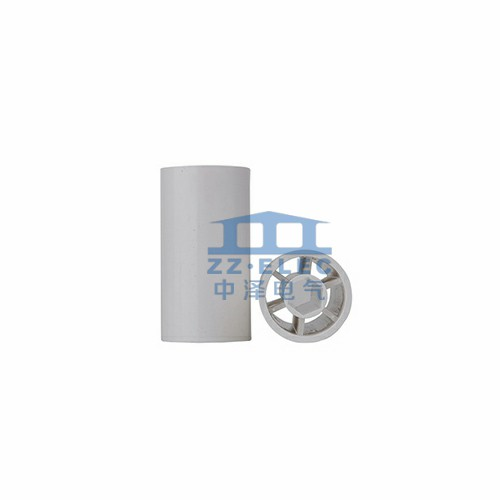# What are the performance indicators of aluminum electrolytic capacitors?

Update:19,Oct,2020
Summary: Performance Nominal capacity and allowable error: the capacity of a capacitor ...
Performance
Nominal capacity and allowable error: the capacity of a capacitor to store electric charge. Commonly used units are F, uF, and pF. The capacitance number marked on the capacitor is the nominal capacity of the capacitor. The nominal capacity of the capacitor and its actual capacity will have an error. Generally, the capacity is written directly on the capacitor, and numbers are also used to mark the capacity. Usually, when the capacity is less than 10000pF, the unit is pF, and when it is greater than 10000pF, the unit is uF. For the sake of simplicity, capacitors larger than 100pF and smaller than 1uF are often not marked with units. If there is no decimal point, its unit is pF, and if there is a decimal point, its unit is uF. If some capacitors are marked with "332" (3300pF) with three significant digits, the first and second digits of the capacitance are given by the two digits from the left, and the third digit indicates the number with 0 after it. The unit It is pF.Rated working voltage: In the specified working temperature range, the capacitor can work reliably for a long time, and the maximum DC voltage it can withstand is the withstand voltage of the capacitor, also called the DC working voltage of the capacitor. If it is in an AC circuit, it should be noted that the maximum value of the AC voltage applied cannot exceed the DC working voltage of the capacitor. Commonly used fixed capacitor working voltages are 6.3V, 10V, 16V, 25V, 50V, 63V, 80V, 100V, 120V, 160V, 200V, 250V, 300V, 350V, 400V, 450V, 500V, 550V, 600V, 630V, 700V, 800V, 1000V.
Insulation resistance: Since the medium between the two poles of the capacitor is not an absolute insulator, its resistance is not infinite, but a finite value, generally above 1000 megohms. The resistance between the two poles of the capacitor is called insulation resistance, or leakage resistance , The size is the ratio of the DC voltage under the rated working voltage to the leakage current through the capacitor. The smaller the leakage resistance, the more serious the leakage. Capacitor leakage will cause energy loss, which not only affects the life of the capacitor, but also affects the operation of the circuit. Therefore, the larger the leakage resistance, the better.
Dielectric loss: the energy consumed by a capacitor under the action of an electric field, usually expressed by the ratio of the loss power to the reactive power of the capacitor, that is, the tangent of the loss angle. The greater the loss angle, the greater the loss of the capacitor, and the capacitor with a large loss angle is not suitable for high-frequency operation.